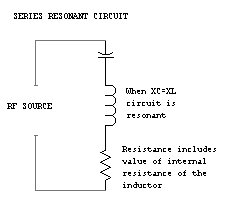SERIES RESONANCE

Introduction | Questions | Quiz
 INTRODUCTION Resonance occurs in a series R-L-C circuit when inductive and capacitive reactances are equal. Resistance does not affect the resonant frequency. Formula for calculating resonant frequency: f = 1 000 000 / 2pi * squarer root of (LC ) f = frequency in kilohertz L = inductance in microhenrys C = capacitance in picofarads 2pi = 6.28 Note: Use calculator to compute values. Look closely at the values of L and C. If the values are small, the resonant frequency is high and all answers in kHz can be eliminated. A-001-003-001.... What is the resonant frequency of a series RLC circuit, if R is 47 ohms, L is 50 microhenrys and C is 40 picofarads? 1.78 MHz 3.56 MHz 7.96 MHz 79.6 MHz A-001-003-002.... What is the resonant frequency of a series RLC circuit, if R is 47 ohms, L is 40 microhenrys and C is 200 picofarads? 1.99 kHz 1.99 MHz 1.78 kHz 1.78 MHz A-001-003-003.... What is the resonant frequency of a series RLC circuit, if R is 47 ohms, L is 50 microhenrys and C is 10 picofarads? 7.12 kHz 3.18 MHz 3.18 kHz 7.12 MHz A-001-003-004.... What is the resonant frequency of a series RLC circuit, if R is 47 ohms, L is 25 microhenrys and C is 10 picofarads? 63.7 MHz 10.1 kHz 63.7 kHz 10.1 MHz A-001-003-005.... What is the resonant frequency of a series RLC circuit, if R is 47 ohms, L is 3 microhenrys and C is 40 picofarads? 13.1 MHz 14.5 MHz 13.1 kHz 14.5 kHz A-001-003-006.... What is the resonant frequency of a series RLC circuit, if R is 47 ohms, L is 4 microhenrys and C is 20 picofarads? 19.9 MHz 17.8 MHz 19.9 kHz 17.8 kHz A-001-003-007.... What is the resonant frequency of a series RLC circuit, if R is 47 ohms, L is 8 microhenrys and C is 7 picofarads? 28.4 MHz 21.3 MHz 2.84 MHz 2.13 MHz A-001-003-008.... What is the resonant frequency of a series RLC circuit, if R is 47 ohms, L is 3 microhenrys and C is 15 picofarads? 35.4 MHz 23.7 MHz 35.4 kHz 23.7 kHz A-001-003-009.... What is the resonant frequency of a series RLC circuit, if R is 47 ohms, L is 4 microhenrys and C is 8 picofarads? 49.7 MHz 28.1 MHz 49.7 kHz 28.1 kHz A-001-003-010.... What is the resonant frequency of a series RLC circuit, if R is 47 ohms, L is 1 microhenry and C is 9 picofarads? 53.1 MHz 5.31 MHz 17.7 MHz 1.77 MHz A-001-003-011.... What is the value of capacitance (C) in a series RLC circuit, if the circuit resonant frequency is 14.25 MHz and L is 2.84 microhenrys? 2.2 microfarads 44 microfarads 44 picofarads 2.2 picofarads QUIZ Try the QUIZ for this topic.HAMSTUDY.COM
Webmaster: Frank VanderZande - VE7AV
Copyright © 2000-2014, Alpha Victor Enterprises Ltd.